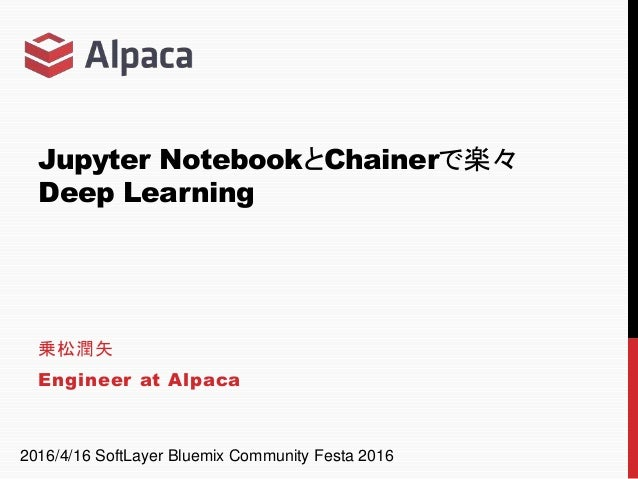Successfully reported this slideshow.Upcoming SlideShare
×

# Jupyter NotebookとChainerで楽々Deep Learning

2016/4/16 SoftLayer Bluemix Community Festa 2016で発表したスライドです。第2部のNotebook -> https://github.com/jnory/slbm16festa_tutorial

• Full Name
Comment goes here.

Are you sure you want to Yes No### Jupyter NotebookとChainerで楽々Deep Learning

1. 1. Jupyter NotebookとChainerで楽々 Deep Learning 乗松潤矢 Engineer at Alpaca 2016/4/16 SoftLayer Bluemix Community Festa 2016
2. 2. 自己紹介 乗松潤矢 (Jun-ya Norimatsu) Engineer @ Alpaca 専門: 自然言語処理 統計的機械翻訳 統計的言語モデル 3月に博士号をとったばかりのペーペーです 2 フリーランス 今日の肩書き
3. 3. Capitalico https://www.capitalico.com/ Deep Learningによる 投資アルゴリズム学習 こんなの作ってます 3
4. 4. Deep Learningで投資アルゴリズム学習? 投資家には、それぞれ思い描いているパターンがある 自分が思っているパターンが来たらお知らせしてほしい 4
5. 5. Deep Learningで投資アルゴリズム学習? これらはすべて同じパターンとして検出したい 実例から機械学習 5
6. 6. 与えられた実例からパターンを学習 6
7. 7. 今日のお話をきくとお得な人たち Deep Learningやってみたいけど腰が重い人 Deep Learning Framework入れてみたけど、「…で？」となった人 過去にやった検証のどれがなにだかわからなくなりがちな人 逆に、時間の無駄になるかもしれない人たち Chainerマスターな人 Jupyter Notebookマスターな人 7
8. 8. 本日の内容 • 第1部 • AlpacaにおけるChainer + Jupyter Notebook環境 • 第2部 • Jupyter NotebookでDeep Learningをやってみる • (Chainer入門) 8
9. 9. Alpacaにおける Jupyter Notebook + Chainer環境 第1部 9
10. 10. 本題の前に… AlpacaにおけるSoftlayerの利用 Alpacaでは開発にDockerをフル活用 Softlayerさまサーバー Docker Webサーバ DBサーバ Jobサーバ Docker Webサーバ DBサーバ Jobサーバ Docker Webサーバ DBサーバ Jobサーバ Aさん用 環境 Bさん用 環境 Cさん用 環境 =container 各環境内で閉じたネットワーク 10
11. 11. 本題の前に… AlpacaにおけるSoftlayerの利用 Softlayerさまサーバー Docker Webサーバ DBサーバ Jobサーバ Docker Webサーバ DBサーバ Jobサーバ Aさん用 環境 Bさん用 環境 =container 一台のサーバーで複数人が同時開発可能 ポイント: Networkがコンテナ内で独立 11Softlayerサーバーをフル活用
12. 12. 本題の前に… AlpacaにおけるSoftlayerの利用 Softlayerさまサーバー Tesla K80 Tesla K80 Docker 12 検証用環境もDockerで構築 Chainer + Jupyter Notebook Docker内からGPUにアクセスできるよう設定
13. 13. 本題 なぜJupyter Notebookなのか 「前にやったあの実験どうなったんだっけ」 「あのとき、ここのパラメータどうしたんだっけ」 「前はうまくいったはずだったんだけど…」 Notebook導入前の悩み 「これから新しく来る人に今までやってきた知見をどう伝えよう」 Notebookのいいところ 実験の手順を残せる コードと一緒に実験結果のグラフ・表を保存できる GitHubにアップロードすると実験結果へのpermalinkができる 13
14. 14. Jupyter Notebook 旧IPython Notebook Pythonだけじゃなくなったので名前変更 ノートブック形式でプログラムを実行・結果を保存 14
15. 15. Notebook形式？ ノートに書き込んでいるかのように対話的なプログラム実行 Markdown Pythonコード 15
16. 16. NotebookファイルをGitHubに上げるとそのまま見える 記録・参照・共有に便利 16
17. 17. Chainer 日本発のDeep Learning Framework Networkをダイナミックに書き換えられる 数あるFrameworkの中で一番書きやすい(※) (※)個人の感想です 17
18. 18. Jupyter Notebook + Chainer Notebook中でimportして使うだけ GPUも問題なく使える 18
19. 19. AlpacaでのJupyter Notebookの利用例 トレーニングの進捗をその場で可視化 training dataに対するlossを可視化 横軸: 正解データの値 縦軸: 推定データの値 training lossの反復に対する変化 横軸: 反復回数 縦軸: lossの値(average) 19
20. 20. AlpacaでのJupyter Notebookの利用例 シグナルの出方をNotebook上で可視化 シグナル 20
21. 21. Notebookの運用(試行錯誤中) タスク管理との連動 NotebookのURL 21
22. 22. Notebookの運用(試行錯誤中) 失敗した実験はどう保存する？ 全部保存していたら大量のNotebookが溢れてしまう (暫定作) Pull Requestを出してマージしない PRから辿ればNotebookを参照できる 22
23. 23. Jupyter Notebook Tips Pythonでやるよりコマンド叩いた方が速いんだけど “%%bash” でbashが使える 23
24. 24. Jupyter Notebook Tips 時間を計りたい “%%time” で実行時間を測定できる 24
25. 25. Jupyter Notebook Tips もっとインタラクティブにグラフを見たいんだけど plotlyが便利 ただしGitHubからは見えなくなる マウスオンで値が見える 拡大できる 25
26. 26. 第1部まとめ • Jupyter NotebookでChainerつかえる • Jupyter Notebookで実験を保存 • その場でグラフ作って結果確認 • GitHubと相性良い • GitHubが実験にpermalinkをくれる • チームメンバー全員が実験結果にアクセス可 • タスク管理ツールと連動すると後から参照しやすい 26
27. 27. Jupyter Notebookで Deep Learningをやってみる (Chainer入門) 第2部 27
28. 28. やってみよう Jupyter Notebookのインストール いろんな方法があります 多分一番楽なのはanaconda chainerのインストール pip install chainer Notebookからpipできる 28
29. 29. Demo 走れメロスっぽい文ジェネレータ 実際に、やってみた Deep Learningで「走れメロス」の文体を学習 学習した文体で文を生成 29
30. 30. 走れメロスっぽい文ジェネレータ 実行例 30
31. 31. 走れメロスっぽい文ジェネレータ うまくいかなかった文 走れメロス本文に出てくる文 なんかおかしい文 31
33. 33. モデルを作ろう やりたいこと: 文を生成したい 生成モデルがいいよね 文(単語列)を とする を与えるモデルがあれば文をサンプリングできる 33
34. 34. よくある式変形 をDeep Learningでモデル化しましょう なので 34
35. 35. 今回のモデル LSTM LSTM Fully Connected EmbedID Softmax Chainerのexampleとほぼ同一 https://github.com/pfnet/chainer/blob/master/examples/ptb 単語を高次元空間の位置に変換 過去の単語と今回の単語を組み合わせて 文脈をベクトル化 ベクトルを確率分布に変換 35
36. 36. 今回のモデル LSTM LSTM Fully Connected EmbedID Softmax LSTM LSTM Fully Connected EmbedID Softmax LSTM LSTM Fully Connected EmbedID Softmax 36
37. 37. Modelを書こう – 全体像 – class LM(Chain): def __init__(self, n_vocab): super(LM, self).__init__( embed=L.EmbedID(n_vocab, 100), lstm1=L.LSTM(100, 50), lstm2=L.LSTM(50, 50), out=L.Linear(50, n_vocab), ) self.n_vocab = n_vocab self.dropout_ratio = 0.5 self.train = False self.softmax = lambda x: x def reset_state(self): self.lstm1.reset_state() self.lstm2.reset_state() def __call__(self, x): h = self.embed(x) h = self.lstm1(h) h = F.dropout(h, ratio=self.dropout_ratio, train=self.train) h = self.lstm2(h) h = F.dropout(h, ratio=self.dropout_ratio, train=self.train) y = self.out(h) y = self.softmax(y) return y LSTM LSTM Fully Connected EmbedID (Softmax) 例えばこんな感じ 37
38. 38. Modelを書こう – レイヤー定義 – class LM(Chain): def __init__(self, n_vocab): super(LM, self).__init__( embed=L.EmbedID(n_vocab, 100), lstm1=L.LSTM(100, 50), lstm2=L.LSTM(50, 50), out=L.Linear(50, n_vocab), ) LSTM LSTM Fully Connected EmbedID (Softmax) パラメータを持つレイヤーの定義 レイヤーはlink.Chainが管理 Point! dropout, softmaxはパラメータを持たない 38
39. 39. Modelを書こう – 確率分布計算 – def __call__(self, x): h = self.embed(x) h = self.lstm1(h) h = F.dropout(h, ratio=self.dropout_ratio, train=self.train) h = self.lstm2(h) h = F.dropout(h, ratio=self.dropout_ratio, train=self.train) y = self.out(h) y = self.softmax(y) return y LSTM LSTM Fully Connected EmbedID (Softmax) レイヤーを順に並べるだけ たまにdropoutを入れるといい感じに学習する Point! 39 (詳細は略)
40. 40. 学習しよう モデルを作っただけではだめ 適切なモデルパラメータを学習しないと使えない モデルパラメータはどうやって学習する？ 学習データをうまく表現するパラメータを探す モデルと学習データのズレ (training) lossと言います lossができるだけ小さくなるようにモデルパラメータを設定 最適化と言います 40
41. 41. 学習しよう モデルパラーメタの学習 lossが小さくなるように モデルのパラメータを少しずつ動かす作業 41
42. 42. 学習 [Step 1] モデルから分類器を作る classifier = links.Classifier(lm) lm = LM(len(vocab)) 次の1単語を予測 正解・不正解を判定 LSTM LSTM Fully Connected EmbedID (Softmax) LM loss classifier( , ) : 「どういう風に当たり/外れたか」を返す 42
43. 43. 変数の取り扱い classifier( , ) ってどう扱う？ って書いたけど、 Variableクラスを使いましょう!! Variable( の単語ID, volatile=...)使い方 volatileに与える値(典型的な使い方の場合) 学習時: False, テスト時: True 43
44. 44. 値の持ち方 通常、複数の文を一度に処理します 文1 文2 文3 … ID(w11) ID(w12) ID(w13) … ID(w1m) ID(w21) ID(w22) ID(w23) … ID(w2m) ID(w31) ID(w32) ID(w33) … ID(w3m) … … … … … 1単語目 2単語目 3単語目 m単語目… ID(w): 単語wの単語IDを返す関数 wij: 文iのj番目の単語, 文長がj未満の場合特殊単語</s> m: データに含まれる文の最大文長+1 このとき、 は Variable(id_vec[:, i], volatile=...) id_vec = 44
45. 45. 学習 [Step 2] lossの計算 学習用データを1回なめる関数 一単語ずつ、予測・当たり/外れ情報が変数lossに溜まっていく 45